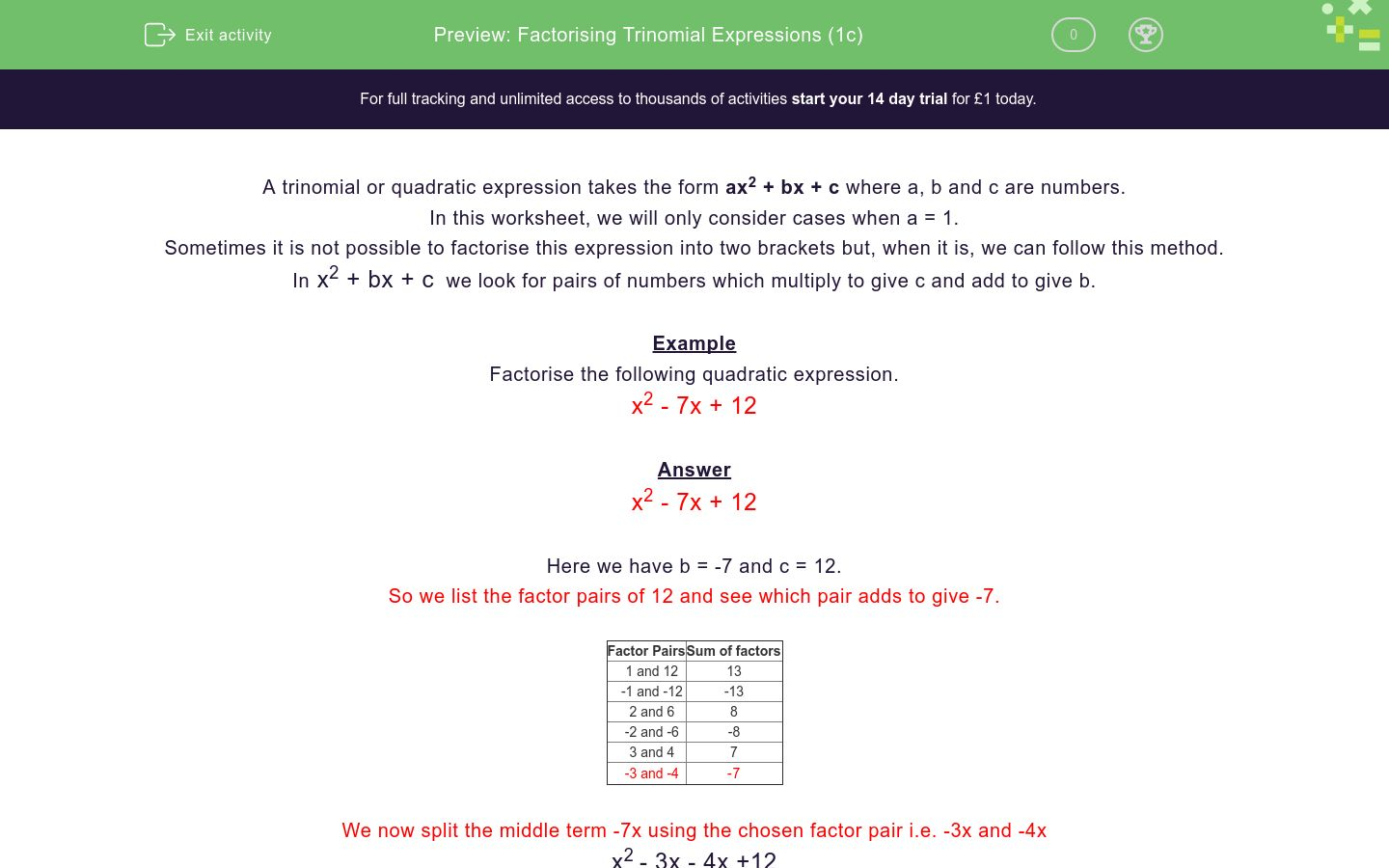# Factorising Trinomial Expressions (1c)

In this worksheet, students factorise simple trinomial expressions.Key stage:  KS 4

Curriculum topic:  Algebra

Curriculum subtopic:  Simplify/Manipulate Algebraic Expressions/Fractions

Difficulty level:### QUESTION 1 of 10

A trinomial or quadratic expression takes the form ax2 + bx + c where a, b and c are numbers.

In this worksheet, we will only consider cases when a = 1.

Sometimes it is not possible to factorise this expression into two brackets but, when it is, we can follow this method.

In x2 + bx + c  we look for pairs of numbers which multiply to give c and add to give b.

Example

Factorise the following quadratic expression.

x2 - 7x + 12

x2 - 7x + 12

Here we have b = -7 and c = 12.

So we list the factor pairs of 12 and see which pair adds to give -7.

Factor Pairs Sum of factors
1 and 12 13
-1 and -12 -13
2 and 6 8
-2 and -6 -8
3 and 4 7
-3 and -4

-7

We now split the middle term -7x using the chosen factor pair i.e. -3x and -4x

x2 - 3x - 4x +12

Consider the expression in pairs

x2 - 3x - 4x + 12

Factorise each pair

x (x - 3) - 4 (x - 3)

Combine

(x - 4) (x - 3)

This is the full factorisation

(x - 4) (x - 3)

Factorise the following quadratic expression.

x2 - 5x + 6

(x - 2) (x - 6)

(x - 6) (x - 1)

(x - 3) (x - 2)

(x - 2) (x - 4)

Factorise the following quadratic expression.

x2 - 7x + 6

(x - 2) (x - 4)

(x - 3) (x - 2)

(x - 1) (x - 6)

(x - 2) (x - 6)

Factorise the following quadratic expression.

x2 - 6x + 8

(x - 2) (x - 4)

(x - 3) (x - 2)

(x - 1) (x - 8)

(x - 2) (x - 6)

Factorise the following quadratic expression.

x2 - 9x + 8

(x + 2) (x - 4)

(x - 3) (x + 2)

(x - 1) (x - 8)

(x - 2) (x - 4)

Factorise the following quadratic expression.

x2 - 6x + 9

(x - 4) (x - 4)

(x - 2) (x - 8)

(x + 1) (x - 9)

(x - 3) (x - 3)

Factorise the following quadratic expression.

x2 - 10x + 9

(x - 4) (x + 4)

(x - 2) (x - 8)

(x - 1) (x - 9)

(x - 3) (x + 3)

Factorise the following quadratic expression.

x2 - 10x + 16

(x - 4) (x - 4)

(x - 2) (x - 8)

(x - 1) (x - 9)

(x + 3) (x - 3)

Factorise the following quadratic expression.

x2 - 8x + 16

(x - 4) (x - 4)

(x - 2) (x - 8)

(x - 1) (x - 9)

(x + 3) (x - 3)

Factorise the following quadratic expression.

x2 - 20x + 36

(x - 9) (x - 4)

(x - 6) (x - 6)

(x - 12) (x - 3)

(x - 18) (x - 2)

Factorise the following quadratic expression.

x2 - 15x + 36

(x - 9) (x - 4)

(x - 6) (x - 6)

(x - 12) (x - 3)

(x - 18) (x - 2)

• Question 1

Factorise the following quadratic expression.

x2 - 5x + 6

(x - 3) (x - 2)
• Question 2

Factorise the following quadratic expression.

x2 - 7x + 6

(x - 1) (x - 6)
• Question 3

Factorise the following quadratic expression.

x2 - 6x + 8

(x - 2) (x - 4)
• Question 4

Factorise the following quadratic expression.

x2 - 9x + 8

(x - 1) (x - 8)
• Question 5

Factorise the following quadratic expression.

x2 - 6x + 9

(x - 3) (x - 3)
• Question 6

Factorise the following quadratic expression.

x2 - 10x + 9

(x - 1) (x - 9)
• Question 7

Factorise the following quadratic expression.

x2 - 10x + 16

(x - 2) (x - 8)
• Question 8

Factorise the following quadratic expression.

x2 - 8x + 16

(x - 4) (x - 4)
• Question 9

Factorise the following quadratic expression.

x2 - 20x + 36

(x - 18) (x - 2)
• Question 10

Factorise the following quadratic expression.

x2 - 15x + 36